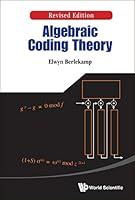#### Algebraic Coding Theory (Revised Edition) (Special Indian Edition / Reprint Year : 2020)

This is the revised edition of Berlekamp's famous book, 'Algebraic Coding Theory', originally published in 1968, wherein he introduced several algorithms which have subsequently dominated engineering practice in this field. One of these is an algorithm for decoding Reed-Solomon and Bose-Chaudhuri-Hocquenghem codes that subsequently became known as the Berlekamp-Massey Algorithm. Another is the Berlekamp algorithm for factoring polynomials over finite fields, whose later extensions and embellishments became widely used in symbolic manipulation systems. Other novel algorithms improved the basic methods for doing various arithmetic operations in finite fields of characteristic two. Other major research contributions in this book included a new class of Lee metric codes, and precise asymptotic results on the number of information symbols in long binary BCH codes.Selected chapters of the book became a standard graduate textbook.Both practicing engineers and scholars will find this book to be of great value.